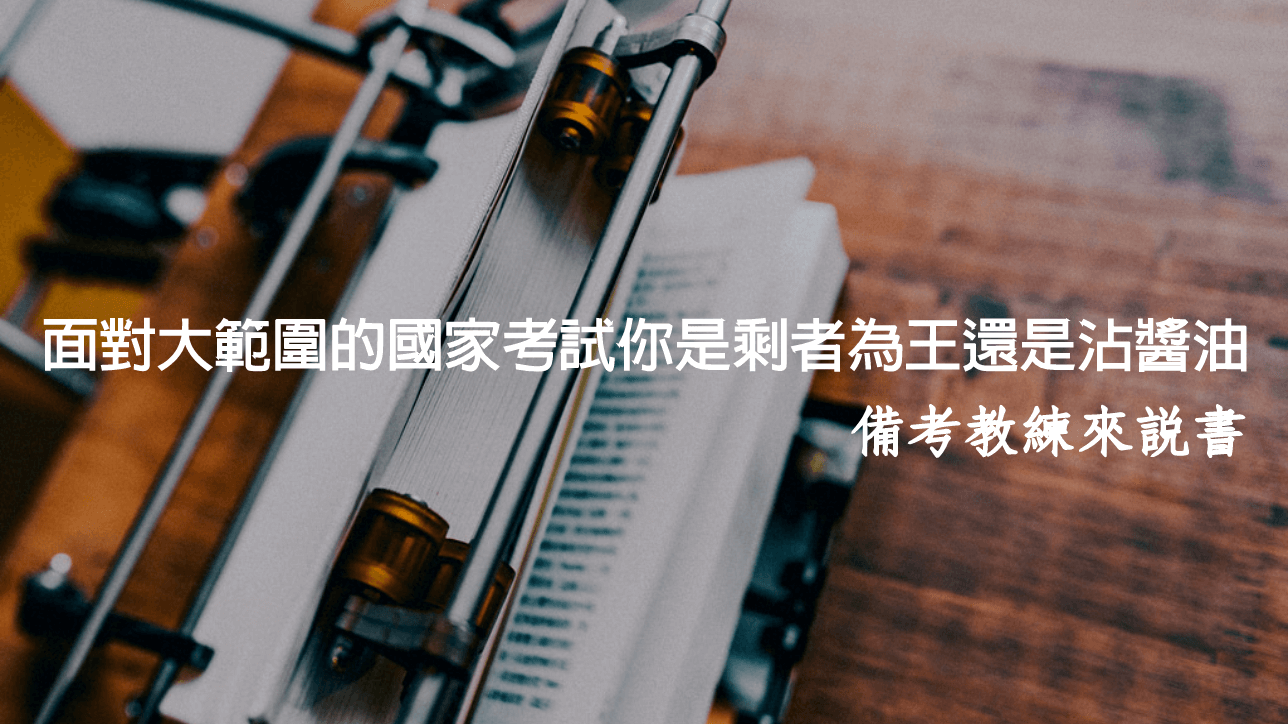「真有這種事?」也許有些人會感到疑惑。

``` (function(i,s,o,g,r,a,m){i['GoogleAnalyticsObject']=r;i[r]=i[r]||function(){ (i[r].q=i[r].q||[]).push(arguments)},i[r].l=1*new Date();a=s.createElement(o), m=s.getElementsByTagName(o);a.async=1;a.src=g;m.parentNode.insertBefore(a,m) })(window,document,'script','//www.google-analytics.com/analytics.js','ga'); ga('create', 'UA-51346956-18', 'auto'); ga('send', 'pageview'); ```
```(function() { var _fbq = window._fbq || (window._fbq = []); if (!_fbq.loaded) { var fbds = document.createElement('script'); fbds.async = true; fbds.src = '//connect.facebook.net/en_US/fbds.js'; var s = document.getElementsByTagName('script'); s.parentNode.insertBefore(fbds, s); _fbq.loaded = true; } })(); window._fbq = window._fbq || []; window._fbq.push(['track', '6026473357653', {'value':'0.00','currency':'TWD'}]); ```
``` (function(i,s,o,g,r,a,m){i['GoogleAnalyticsObject']=r;i[r]=i[r]||function(){ (i[r].q=i[r].q||[]).push(arguments)},i[r].l=1*new Date();a=s.createElement(o), m=s.getElementsByTagName(o);a.async=1;a.src=g;m.parentNode.insertBefore(a,m) })(window,document,'script','//www.google-analytics.com/analytics.js','ga'); ga('create', 'UA-51346956-15', 'auto'); ga('send', 'pageview'); ``` ```(function() { var _fbq = window._fbq || (window._fbq = []); if (!_fbq.loaded) { var fbds = document.createElement('script'); fbds.async = true; fbds.src = '//connect.facebook.net/en_US/fbds.js'; var s = document.getElementsByTagName('script'); s.parentNode.insertBefore(fbds, s); _fbq.loaded = true; } })(); window._fbq = window._fbq || []; window._fbq.push(['track', '6026473357653', {'value':'0.00','currency':'TWD'}]); ```
``` (function(i,s,o,g,r,a,m){i['GoogleAnalyticsObject']=r;i[r]=i[r]||function(){ (i[r].q=i[r].q||[]).push(arguments)},i[r].l=1*new Date();a=s.createElement(o), m=s.getElementsByTagName(o);a.async=1;a.src=g;m.parentNode.insertBefore(a,m) })(window,document,'script','//www.google-analytics.com/analytics.js','ga'); ga('create', 'UA-51346956-15', 'auto'); ga('send', 'pageview'); `````` (function(i,s,o,g,r,a,m){i['GoogleAnalyticsObject']=r;i[r]=i[r]||function(){ (i[r].q=i[r].q||[]).push(arguments)},i[r].l=1*new Date();a=s.createElement(o), m=s.getElementsByTagName(o);a.async=1;a.src=g;m.parentNode.insertBefore(a,m) })(window,document,'script','//www.google-analytics.com/analytics.js','ga'); ga('create', 'UA-51346956-18', 'auto'); ga('send', 'pageview'); ```

【★最新備考策略活動★】走在備考道路上，不少準備考試未滿半年或者備考超過一年以上的考生，時常感到迷惘，甚至找不到合適自己備考方法而痛苦著，面對公職考試其實是一連串的選擇，只要做出一次的更好的選擇，備考命運就會改寫。備考教練團隊梳理上千位考生常遇到的備考困擾，希望協助已下定決心❶購買書籍❷函授課程或者❸參與補習班實體課程的考生，對大範圍備考有概念，藉此能夠清楚知道自己的問題在哪裡、需要解決的是什麼，想要玩贏這場備考遊戲嗎？不妨借力備考教練團隊，幫你的備考模式一步一步地來診斷，找出病因並對症下藥，現在請點擊連結➦https://bit.ly/323X5w7咱們專屬頁面中見